3. CURRENT ELECTRICITY

Q.1. What do you mean by colour code of carbon resistance ?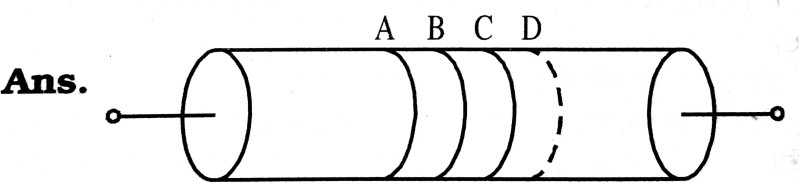The value of resistances used in electric and electronic circuits vary over a large range. Such resistances are marked on them according to colour code. These high resistances are generally carbon resistances which have four bands of different colours named as A, B, C, D. The first two bands A and B represent first two significant figures of the value of resistance (in Ω). The third band C represents the decimal multiplier and fourth band D gives the tolerance of the resistance known as the limit of accuracy or the permitted variation in the value of resistance. The colour code can be remembered with follow sentence :
0    1   2    3   4   5            6                       7           8           9
B  R  O  Y  Great  Britain  has  Very  Good  Wife.

 Letter Colour Figure Multiplier Tolerance and Colour B Black 0 100 Gold = ± 5% Silver = ± 10% No colour = ± 20% B Brown 1 101 R Red 2 102 O Orange 3 103 Y Yellow 4 104 G Green 5 105 B Blue 6 106 V Violet 7 107 G Grey 8 108 W White 9 109

Q.2. A given wire is stretched to reduce its diameter to half its previous value. What will be its new resistance ?

Ans ⇒ Let D be the original diameter of the wire,then diar
New area of the wire is given by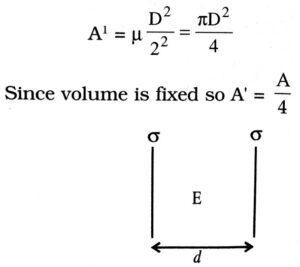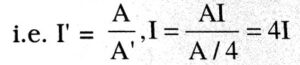if area is decreased to one fourth then wire can be stretched 4 times.
Now, new resistance,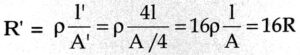New resistance will be sixteen times the original resistance.

Q.3. Define the terms resistivity, conductivity and state their SI unit. Draw a graph showing the variation of resistivity with temperature for a typical semiconductor.

Ans ⇒ Resistivity : The resistance of a material of a conductor of unit length and unit area of cross section.
SI unit : Wm
Conductivity : The reciprocal of resistivity of the material, It is presented by SI Unit: Ohm-1 m-1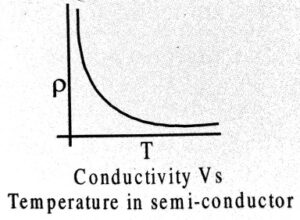Q.4. State the principle of potentiometer. Draw a circuit diagram used to compare the e.m.f. of two primary cells.

Ans ⇒ Principle : If a constant current is passed through a wire of uniform area of cross section, the potential drop across any portion of the wire is directly proportional to the length of the portion. Mathe matically, V α I

Formula used : If E1 and E2 represent the EMF of the cells and I1 and l2 represent the lengths from position A, at which the null point is achieved, then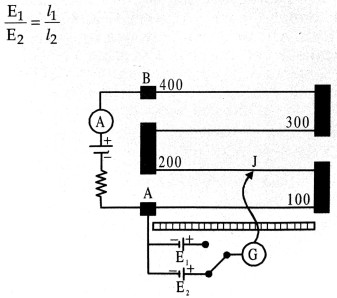Sensitivity of the potentiometer can be increased by increasing the length of the potentiometer wire, 1.e., by decreasing the potential drop per unit length of the wire.

Q.5. Draw the graphs showing variation of inductive reactance and capacitive reactance with frequency of applied AC source, Can the voltage drop across the inductor or the capacitor in a series LCR circuit be greater than the applied voltage of the AC source ?

Ans ⇒ Inductive reactance α ω
Capacitive reactance α1/ω
Yes, the voltage drop across XL, or XC, can be greater than the supply voltage, however, the vector sum of the voltage components should be equal to the net supply voltage itself.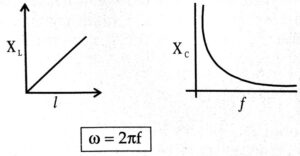Q.6. Write the principle of potentiometer. The variation of potential difference V with length in the case of two potentiometers x and y is shown in figure. Which one of these two will you prefer for comparing emfs of two cells and why?

Ans ⇒ Principle of Potentiometer:The potential difference across any length of the wire of a uniform cross-section and uniform composition is proportional to its length when a constant current flows through it represents a smaller potential gradient. Smaller the potential gradient more is the sensitivity of the potentiometer.

Q.7. An enclosed battery consists of 12 identical cells in series. It is suspected that some of the cells are incorrectly connected. The battery is connected in series with an ampere meter and 2 additional identical cells. When the additional cells support the battery, How many cells are wrongly connected ?

Ans ⇒ Let e be the emf of each cell and E the emf of the battery, then as shown in circuit diagram (Fig)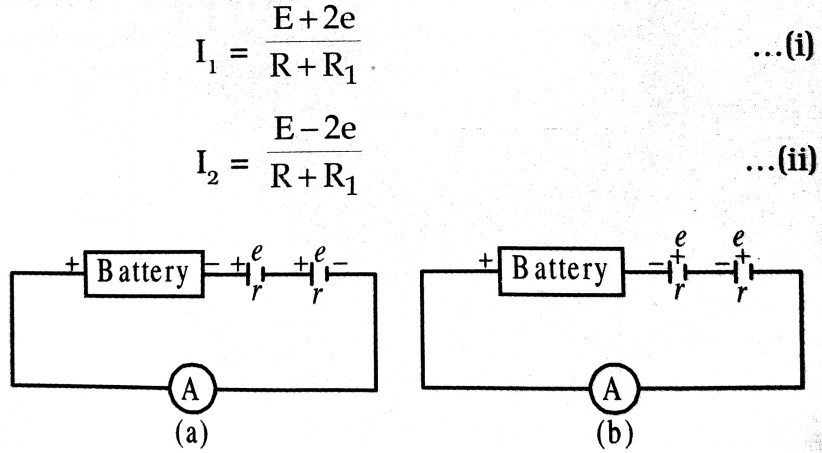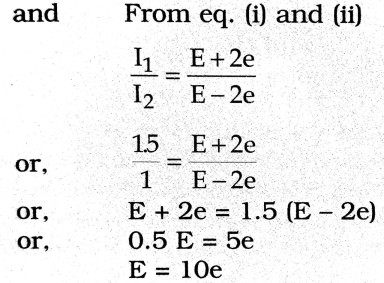The emf of the battery is equivalent to the emf of 10 cells, that is, one cell is wrongly connected.

Q.8. Write the mathematical relation between mobility and drift velocity of charge carriers in a conductor. Name the mobile charge carriers responsible for conduction of electric current in (i) an electrolyte (ii) an ionized gas.

Ans ⇒ The relation between mobility and drift velocity of charge carriers in the conductor is given by
μ= Vd/E
Mobile charge carriers in
(i) In electrolyte – positive and negative ions.
(ii) In ionised gas – positive charge ions.

Q.9. You are given n resistors each of resistance r. These are first connected to get minimum possible resistance. In the second case these are again connected differently to get maximum positive resistance, Compute the ratio between the minimum and maximum value of resistance so obtained.

Ans ⇒ To get minimum resistance, we connect them in parallel i.e., rp = r/n
To get maximum resistance, we connect them in series i.e. rp = nr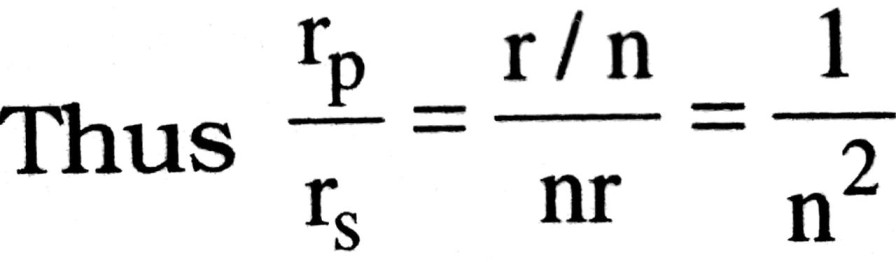Q.10. Draw V-I graph for ohmic and non-ohmic materials, Give one example for each.

Ans ⇒ V-I graph for an ohmic material is a straight line passing through origin Fig. (a) Example a resistor made of manganin. V-I graph for a non-ohmic material is a curve i.e, non-linear. [Fig. (b)] Example is electrolyte of junction diode.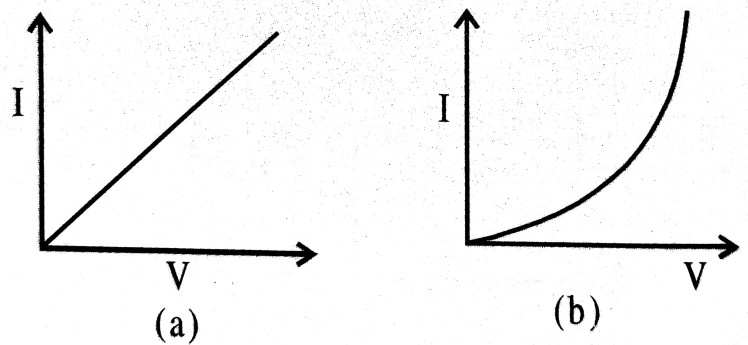Q.11. How does the resistivity of (i) a conductor and (ii) a semiconductor vary with temperature ? Give reason for each case.

Ans ⇒ (i) Resistivity, ρ = m/ne2τ
On increasing temperature collision of free electrons with ions/atoms increases. Thus, relaxation time decreases. According to the relation resistivity increases with increase in temperature.
As ‘n’ does not change appreciably it will not affect resistivity.

(ii) In semiconductors charge in number density of free electrons (n) is sufficient to decrease the resistivity. As ‘n’ was low initially collision do not increases appreciably. Hence relaxation time does not affect much.

Q.12. Establish a relation between current and drift velocity.

Ans ⇒ Consider a conductor ‘1’ and area of cross section ‘A’. If ‘n’ is number density of free electrons, then total charge on all the free electrons in the conductor is Q = Al ne on applying a constant potential difference V across the conductor an electric field is set up.
E= V/I

This electric field makes the charges move. Electrons begin to move with drift velocity V. in a direction oppositve to that of the field. Time taken by free electrons to cross the conductor is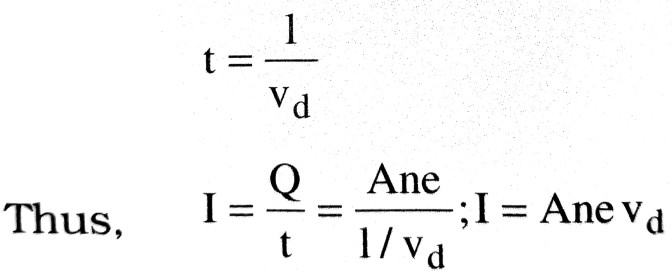Q.13. What is terminal potential difference of a cell ? Can its value be greater than the e.m.f. of a cell ? Explain.

Ans ⇒ Terminal potential difference of a cell is defined as the potential difference between the two electrodes of a cell in a closed circuit. The value of terminal potential difference of a cell is less than the e.m.f. of a cell, when current is drawn from the cell (i.e., during discharging of cell). The value of terminal potential difference of a cell becomes greater than the e.m.f. of the cell during charging of the cell, i.e. the positive electrode of the cell is connected to positive terminal of battery charger and negative electrode of the cell is connected to negative terminal of battery charger.

Q.14. Define the term current density of a metallic conductor. Deduce the relation connecting current density (J) and the conduc tivity (σ) of the conductor, when an electric field (E) is applied to it.

Ans ⇒ Current density of a metallic conductor is defined as the amount of current flowing per unit area of the conductor around that point provided the area is held in a direction normal to the current.
We know, I = ne Avd.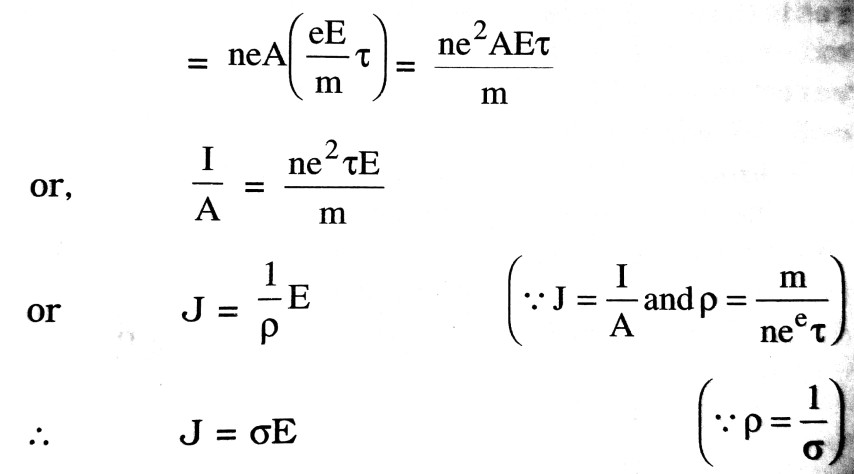Q.15. What are thermistors ? Explain its use in brief.

Ans ⇒ Thermistor is a heat sensitive device whose resistivity changes very rapidly with change of temperature. The temperature coefficient of resistivity of a thermistor is very high, which may be positive or negative. A thermistor can have a resistance in the range of 0.1 Ω. to 107 Ω Thermistors are used (i) to detect small temperature changed (ii) to safe guard the filament of picture tube of a television set against the variation of current (iii) in temperature control units of industry (iv) for voltage stabilisation and remote sensing.

Q.16. Under the conditions will the strength of current in a wire be the same for connection in series and in parallel of n identical cells ?

Ans ⇒ When n cells are connected in series;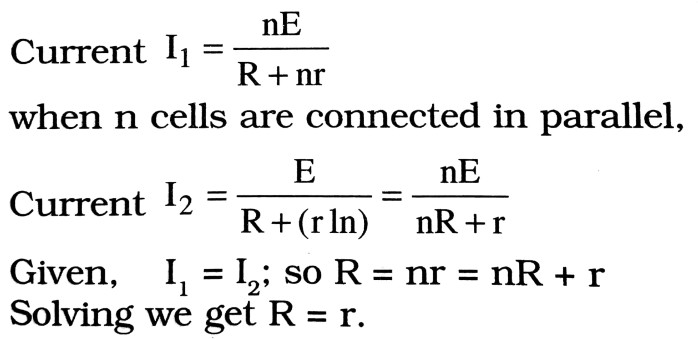Q.17. If the length of the wire be (i) doubled and (ii) halved, what will be the effect on the position of zero deflection in a potentiometer ? Explain.

Ans ⇒ (i) When length of the wire is doubled. The potential gradient across the potentiometer wire will decrease. Due to which the position of zero deflection will occur at longer length.
(ii) The reverse will be the case at first.

Q.18. Define the term ‘temperature coefficient of resistivity’. Write its S.I. unit and plot a graph showing the variation of resistivity of copper with temperature.

Ans ⇒ Temperature coefficient of resistivity : The temperature coefficient of resistivity is defined as the change in resistivity per unit original resistivity per unit change in temperature. S.I. unit is k-1.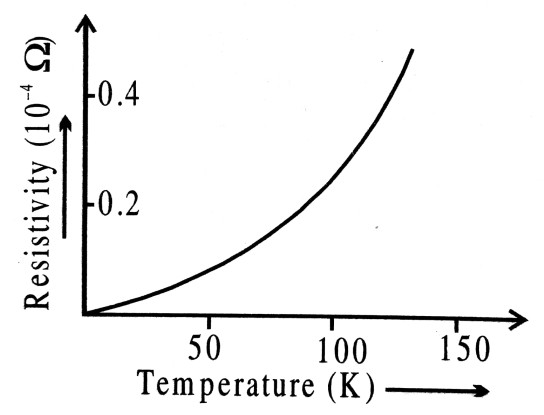The Fig. shows the variation of resistivity of copper with temperature.

Q.19. How can you make a potentiometer of a given length more sensitive by using a resistance box ?

Ans ⇒ The sensitivity of a potentiometer is the smallest potential difference it can measure. It can be increased by reducing the potential gradient i.e. potential drop per unit length of potentiometer wire. The same is possible by decreasing the current flowing in the potentiometer wire, which turn can be achieved by using more resistance from resistance box in series of potentiometer wire.

Q.20. Define the term electrical resistivity of a material. Write its S.I. units. Derive an expression for the resistivity of a metal in terms of number density and mass of free electrons present in it.

Ans ⇒ Electrical resistivity of a material of conductor is the resistance offered by unit length and unit area of cross-section of a wire of the material. Its S.I. unit is Ωm.
The drift velocity of free electrons in a conductor is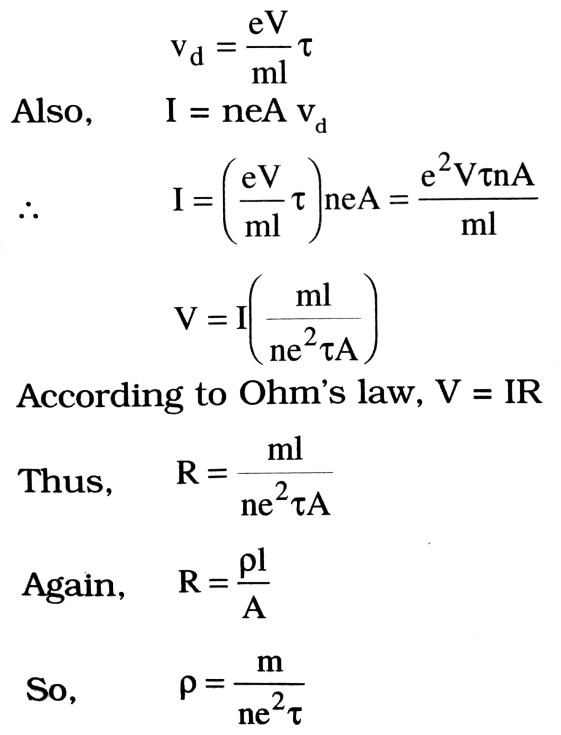Q.21. A toaster produces more heat than a light bulb when they are connected in parallel. Which has the greater resistance ?

Ans ⇒ Since the toaster and the light bulb are connected in parallel, the potential difference cross each is the same (say V).

Let R1 R2 = resistance of toaster and bulb respectively. Amount of heat produced per second by toaster = V2 / R1. Amount of heat produced per second by lamp V2R2. Since toaster produces more heat than the bulb.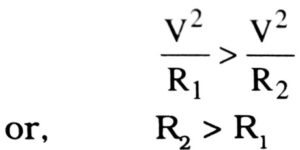Thus the resistance of lamp (R2) is greater than that of a toaster (R1).

Q.22. Using theory of drift velocity, express Ohm’s law.

Ans ⇒ If the length of conductor is L and p.d. is = V
then E = V/I                    …(i)
If the drift velocity of electron is Vd then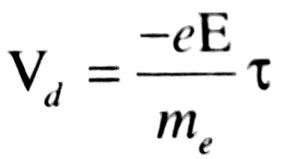…(ii)
where e = charge of electron and τ is relaxation time.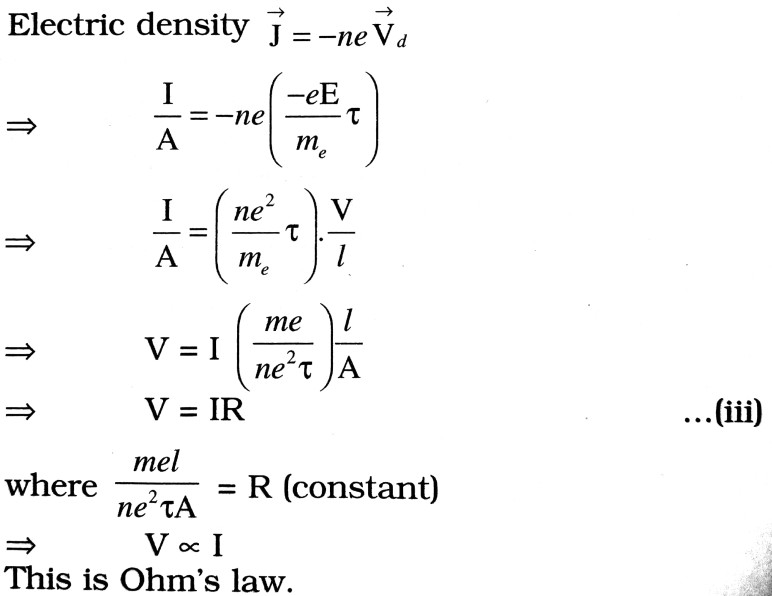Q.23. Explain that second law of Kirchoff is the law of conservation of energy.

Ans ⇒ According to Kirchoff’s second law in a closed network of conductors the sum of the product of current and resistance of each branch of the network is equal to the total e.m.f. of the network. Since the product of current and resistance is equal to potential difference (V = IR). Hence the total e.m.f. supplied in the networks is utilised in the form of potential drop (p.d.) in each branch of the network.

## Class 12th physics Subjective question in English

 S.N Physics Short Type Question English Medium 1. ELECTRIC CHARGES AND FIELDS 2. LECTROSTATIC POTENTIAL AND CAPACITANCE 3. CURRENT ELECTRICITY 4. MOVING CHARGES AND MAGNETISM 5. MAGNETISM AND MATTER 6. ELECTROMAGNETIC INDUCTION 7. ALTERNATING CURRENT 8. ELECTROMAGNETIC WAVES 9. RAY OPTICS AND OPTICAL INSTRUMENTS 10. WAVE OPTICS 11. DUAL NATURE OF MATTER AND RADIATION 12. ATOMS 13. NUCLEI 14. SEMI CONDUCTOR ELECTRONICS 15. COMMUNICATION SYSTEMS